Support Union Test Prep

Support Now

# Page 1-Data Analysis and Statistics Study Guide for the Math Basics

## How to Prepare for the Data Analysis and Statistics Questions on a Math Test

### General Information

With the ability to gather seemingly endless information due to the internet, we need to know how to process the data. At the basic level, that’s all Data Analysis and Statistics is: the collecting, processing, organizing, and modeling of information. It’s becoming increasingly important to know these skills not only in the workplace, but also as a savvy consumer of advertised products and information. So let’s get started!

### Basic Vocabulary

If you’re trying to understand how many people, animals, or objects behave as a group, it’s best to use a measure of central tendency to describe the behavior. For all three measures of central tendency below, let’s analyze the set of grades you’ve had on 10 different math tests:

$\{85,\;73,\; 99,\; 80,\; 82,\; 90,\; 93,\; 77,\; 84,\; 90\}$

#### Mean

Usually, your math teacher will use this measure of central tendency to calculate your final grade in the class. The mean is just the average. To find the mean, take the sum of all the values and divide it by the total number of tests.

$mean= \dfrac{\text{sum of values}}{\text{number of values}}$

So, let’s find the average (or mean) of the test scores.

$mean = \dfrac{85+73+99+80+82+90+93+77+84+90}{10\;tests}$ $mean = \dfrac{853}{10}$ $mean = 85.3$

#### Median

The median is just the middle value of a data set. To find the median, the first step is to order the data from least to greatest:

$\{73,\;77,\;80,\;82,\;84,\;85,\;90,\;90,\;93,\;99\}$

Now, find the middle number by crossing out the highest and lowest values and working your way in.

$$\require{enclose}$$ $$\require{cancel}$$

$\{ \cancel{73},\;77,\;80,\;82,\;84,\;85,\;90,\;90,\;93,\;\cancel{99}\}$ $\{ \cancel{73},\;\cancel{77},\;80,\;82,\;84,\;85,\;90,\;90,\;\cancel{93},\;\cancel{99}\}$ $\{ \cancel{73},\;\cancel{77},\;\cancel{80},\;82,\;84,\;85,\;90,\;\cancel{90},\;\cancel{93},\;\cancel{99}\}$ $\{ \cancel{73},\;\cancel{77},\;\cancel{80},\;\cancel{82},\;84,\;85,\;\cancel{90},\;\cancel{90},\;\cancel{93},\;\cancel{99}\}$

Notice, there are two middle numbers:

$\{ \cancel{73},\;\cancel{77},\;\cancel{80},\;\cancel{82},\;\enclose{circle}{84},\;\enclose{circle}{85},\;\cancel{90},\;\cancel{90},\;\cancel{93},\;\cancel{99}\}$

If this happens (which it will every time there are an even number of values), find the average of the two middle numbers:

$median=\dfrac{84+85}{2}=\dfrac{169}{2}=84.5$

NOTE: You don’t have to do this if there are an odd number of values. Let’s look at this set:

$\{1,\; 4,\; 5,\; 8, \; 10 \}$

Now start crossing out and you’ll arrive at just one middle number: $$5$$.

$$\require{cancel}$$ $$\require{enclose}$$

$\{\cancel{1},\; \cancel{4},\; \enclose{circle}{5},\; \cancel{8}, \; \cancel{10} \}$

#### Mode

The mode is the easiest of the $$3$$ measures of central tendency to find. The mode is just the most often occuring value. In this case, $$90$$ occurs twice, so $$90$$ is the mode.

If all values occur the same number of times, we say there is no mode.

Occasionally, two or more values might occur more than the others, like in the following set:

$\{ 4, \; 4, \; 5, \; 8, \; 8, \; 9, \; 10\}$

In this case, both $$4$$ and $$8$$ are modes.

### Types of Graphs

In order to make data meaningful, we’ve found ways to express the data as a type of graph. They can be used to show trends, comparisons, or the bigger picture and are often easier to use than lists of data.

#### Circle Graph

Circle graphs (or pie charts) are useful when showing percentages. Pie charts help to put things in perspective. Bigger pieces of the pie represent higher percentages. In this example, the whole circle represents the United States Federal Spending in the 2017 fiscal year. You can see from a glance that the most money was spent on health care, pensions, and defense. Look a little closer to find the actual percentages.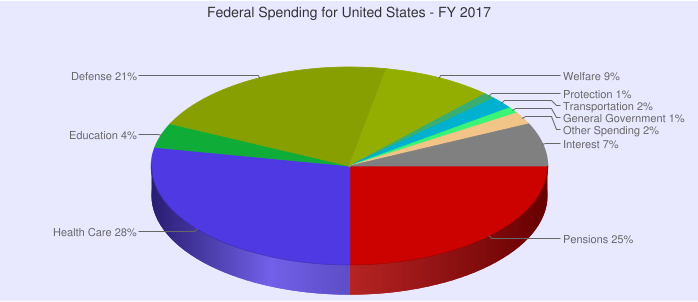When making an accurate circle graph, you’ll need to first calculate percentages. Remember,

$Percent = \dfrac{part}{whole} \cdot 100$

Then, you’ll need to draw a piece of the pie to represent it. For a quick reference, know that $$25\%$$ takes up $$\frac{1}{4}$$ of the circle, or a $$90^\circ$$ angle.

If you need to find the exact angle, use this formula:

$Angle = \dfrac{percent}{100} \cdot 360^\circ$

#### Bar Graph

Bar graphs or charts can be used almost any time you would use a circle chart. They can be arranged vertically or horizontally. Each bar corresponds to an actual amount instead of a percentage.

Horizontal Bar Chart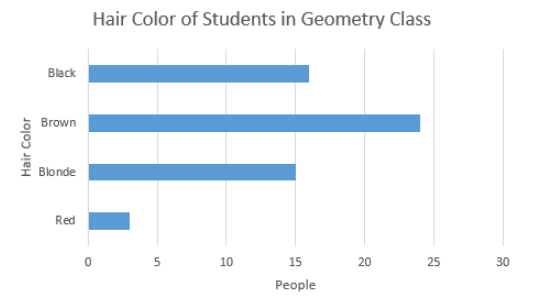Vertical Bar Chart

Occasionally, the actual value is placed at the tip of the bar to make it easier for the reader.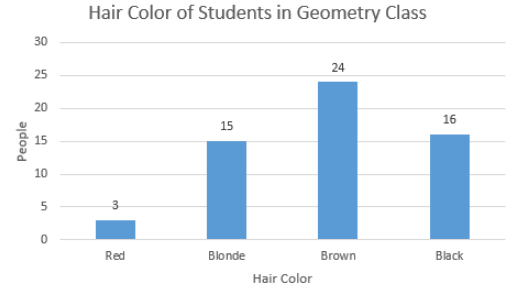#### Line Graph

Line graphs are composed of two axes. The bottom axis is usually time (years, months, days, etc). They are useful in showing trends of data. For example note this graph seems to show that as time goes on, US spending is increasing.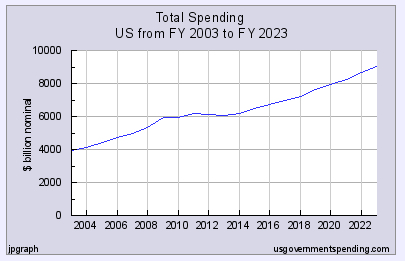Line graphs are made by collecting data every so often. In this case, it was every year. Dots are put on each piece of data. In 2010, for instance, the government spent 6000 billion dollars. Once all the dots are drawn, connect them from left to right. Sometimes graph makers choose to keep the dots present, otherwise, they blend them into the line.

How much money was spent in 2016? Move over to 2016 on the bottom axis. The line above it has a height of just under 7000 billion dollars.

#### Scatterplot

A scatterplot is composed of two axes. Each piece of data is represented as a dot on the plane.

Here’s an example comparing heights and weights of various people.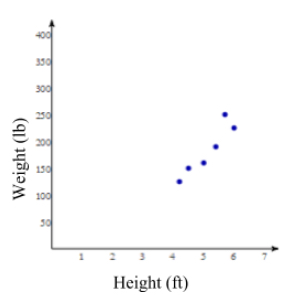Read the data the same way you’d read points on the x-y plane. For example, you can see that there is a 4 ft person who weighs roughly 125 pounds (see the lower left dot). In total, there are 6 people who submitted their weights and heights.

#### Histogram

A basic histogram is basically a line chart mixed with a bar graph. Two axes again, usually with time on the bottom. Dots are made for each date. Instead of drawing lines connecting dots, draw a bar going up to the dot.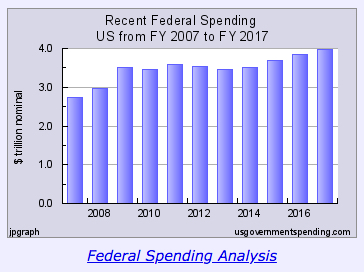Sometimes, you can make more interesting histograms. You can break up the bars similar to the way you’d break up the pieces of a pie chart. In the case below, the author wants to show that the combined total money spent on housing, food, and clothing has been declining over time. In addition, each bar has been split up to show how each particular amount has changed over time. At a glance, it looks like the clothing and food sections have decreased over time while housing has fluctuated.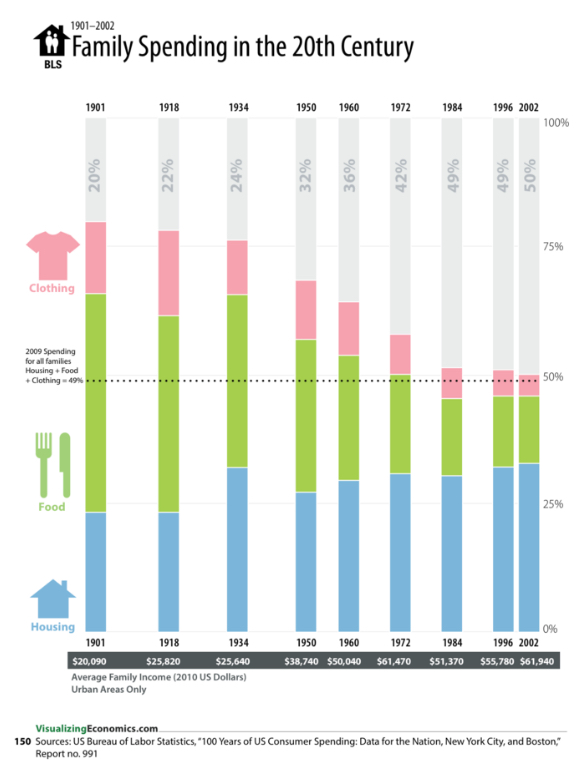#### Box Plot

To create a box plot or box and whisker plot you need to find $$5$$ things about your data set: minimum, first quartile, median, third quartile, and maximum.

Start by ordering your data. In this example let’s look at a few test scores in a class:

$\{45,\; 75, \; 76, \; 78, \;80, \; 82, \; 82, \;85, \; 90, \;92, \;95, \;99 \}$

The minimum is the smallest number: $$45$$

The maximum is the largest number: $$99$$

The median is the middle number. In this case, both 82s are the middle, so the average of them is 82.

Now, split the set right in the middle into two sets.

$\{45,\; 75, \; 76, \; 78, \;80, \; 82, \|\; 82, \;85, \; 90, \;92, \;95, \;99 \}$

The lower half: $$\{45,\; 75, \; 76, \; 78, \;80, \; 82\}$$

And the upper half: $$\{ 82, \;85, \; 90, \;92, \;95, \;99 \}$$

The first quartile or Q1 is the median of the lower half: $$77$$ (the average of $$76$$ and $$78$$)

The third quartile or Q3 is the median of the upper half: $$91$$ (the average of $$90$$ and $$92$$)

Now that you have all five numbers, everything between Q1 and Q3 is enclosed in a box. Then, the whiskers extend to the minimum and maximum.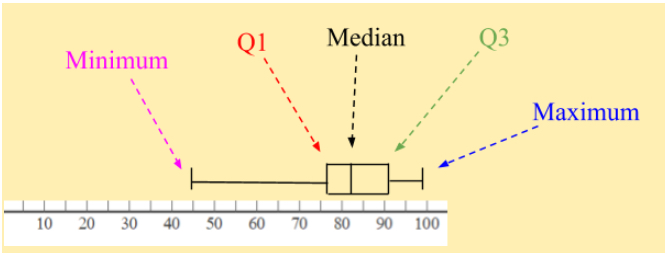You can calculate the range of data by subtracting the minimum from the maximum.

$\text{Range} = \text{Maximum} - \text{Minimum}=99-45=54$

You can also find the interquartile range or IQR by subtracting Q1 from Q3.

$IQR = Q3-Q1=91-77=14$

## All Study Guides for the Math Basics are now available as downloadable PDFs

View other purchase options